Author: Oscar Cronquist Article last updated on December 03, 2019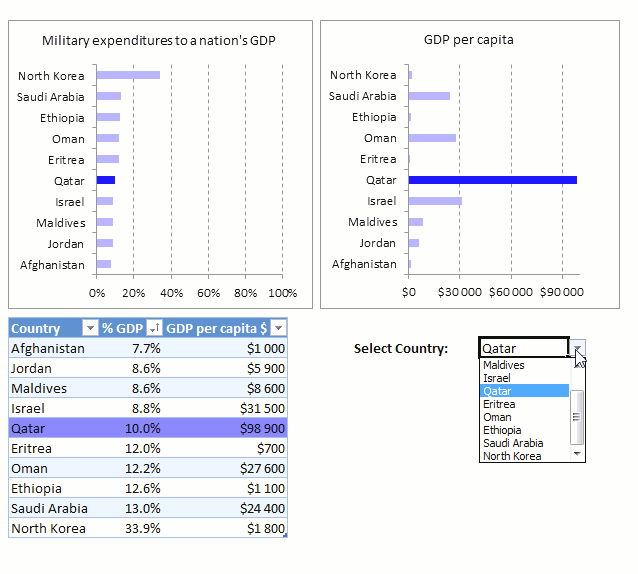This article demonstrates how to highlight a bar in a chart, it allows you to quickly bring attention to a specific chart bar in a chart. Highlighted bars in two or more charts make it easier to read and make comparisons.

This example has a Drop Down List that lets the user choose a value from a list. The list is expanded when you click the arrow next to the cell, make sure you have the cell selected if you don't see the arrow.

I recommend that you show the user that a certain cell contains a drop down list, for example, create a border around the cell or change the cell color.

So what happens when the user selects a value in the drop down list?

Both the charts above the drop down list highlight instantly the corresponding bar and the Excel Table to the left of the drop down list highlights the record.

### Highlight a bar in a chart

The first part of this article explains how to highlight a chart bar with only formulas and a drop down list.

We will start with how to create an Excel Table then copy the Table and paste to a new location. The copied Table will be different from the first Table, it will contain formulas that change based on the selected value in the drop down list.

The bar chart will be linked to both these Excel defined Tables, one chart series to the first Excel Table and the second chart series to the second Excel Table that contains formulas.

#### Create an Excel table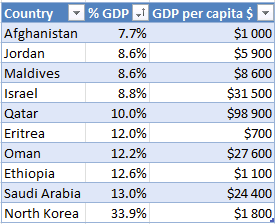The Excel defined Table is great in many ways, the biggest advantage, in my opinion, is combined with a chart you don't need to change the data source reference when new values are added or deleted.

The chart refreshes instantly if new values are added which makes it a great combo, here are the steps to create an Excel Table.

1. Select the cell range that will be the chart data source.
2. Press CTRL + T to open the "Create Excel Table" dialog box.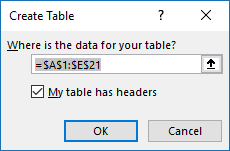3. Click on OK button to create the Excel Table.

#### Copy Excel Table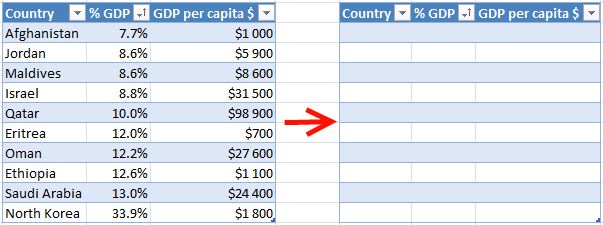Select the entire first Excel Table then copy it, I usually press CTRL + C to copy things in Excel which is the keyboard shortcut for copying.

Select a new cell where you want to paste it, remember to not select an adjacent cell to the copied Excel Table, however, it must be next to the original Table meaning it must be on the same rows.

Press CTRL + V to paste the Excel Table to the new location, this will create a new Excel Table identical to the first one, except for the Excel Table name. Two Excel Tables can't share the same name.

Now delete all values except headers in the new Excel Table, see image above.

#### Create a bar chart

1. Select Excel Table columns Country and % GDP in the first Excel Table.
2. Go to tab "Insert" on the ribbon.
3. Click on "Clustered Bar" button.

The chart shows up on the worksheet, see image below.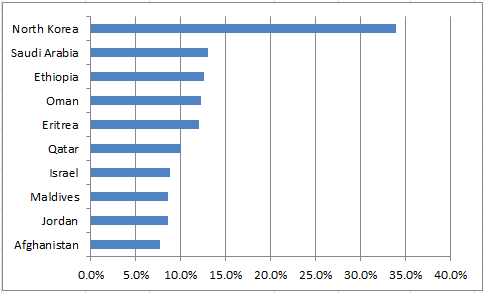#### Create a drop down list

1. Select a cell (H18)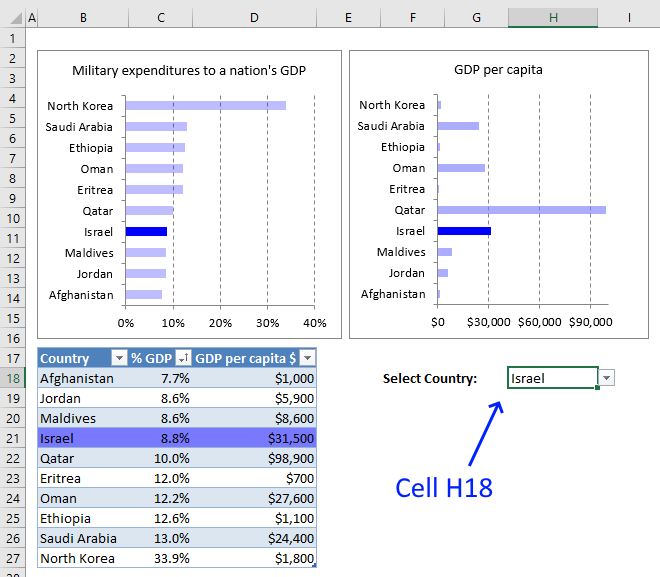2. Go to tab "Data" on the ribbon.
3. Click "Data validation" button.
4. Click "Data Validation..." and a dialog box appears, see image below.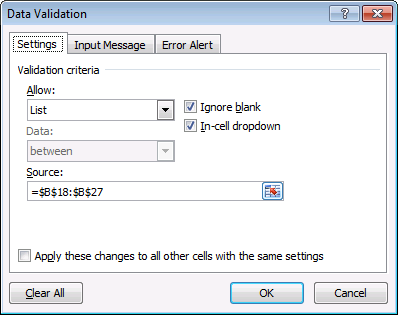5. Change to Allow: List
6. Source: =INDIRECT("Table13[Country]")
7. Click OK button to apply changes.

The Drop Down List contains all countries entered in the Excel Table, it will automatically add new countries. No need to adjust cell ranges, the Excel Table uses structured references which are dynamic.

The INDIRECT function lets you use structured references in Drop Down Lists.

#### Enter formulas in empty Excel Table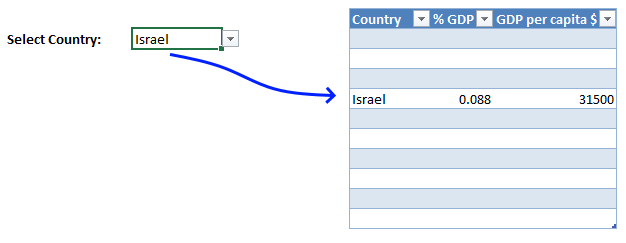The formulas described below will show the selected record based on the value in the Drop Down List, see image above.

1. Select the first cell in table column Country
2. Type
=IF(Table13[@Country]=\$H\$18,Table13[@Country],"")

\$H\$18 is the cell reference to the Drop Down List.

3. Press Enter which will automatically fill the cells below with the same formula.
4. Select the first cell in table column % GDP Type
=IF(Table13[@Country]=\$H\$18,Table13[@[% GDP]],"")
5. Press Enter.
6. Select the first cell in table column "GDP per capita \$"
7. Type
=IF(Table13[@Country]=\$H\$18,Table13[@[GDP per capita \$]],"")
8. Press Enter.

These IF functions will check the selected Drop Down List value with the Country on the same row as the formula, this is why it is important to place the Excel Table with formulas on the same rows as the original Excel Table.

The record will be visible if the Drop Down List value matches the country.

#### Add a duplicate series to the secondary axis

1. Right click on chart.
2. Click "Select Data...".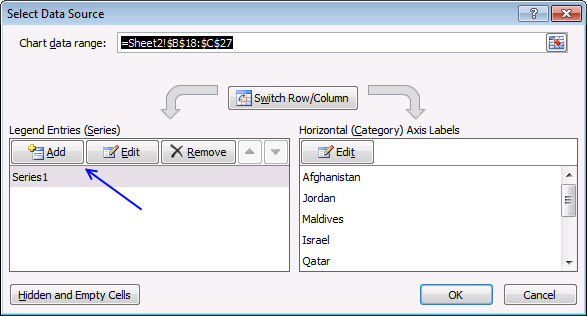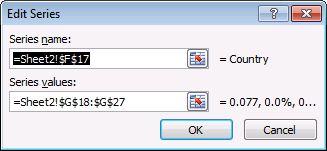4. Series name: Cell reference to table column header "% GDP".
5. Series values: Cell reference to table column "% GDP".
6. Click OK button.
7. Click OK button.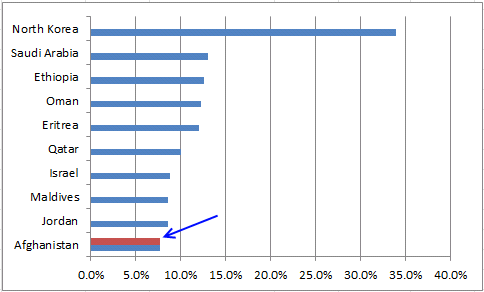8. Select the new series on the chart.
9. Right click on series.
10. Click "Format Data Series...".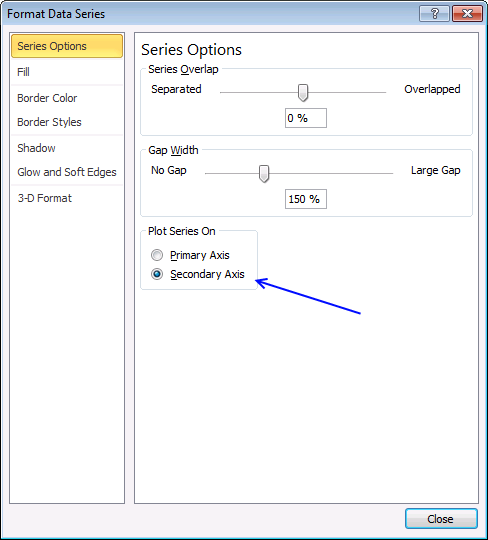11. Select the secondary axis.
12. Click Close button.

#### Delete axis

1. Select duplicate x axis.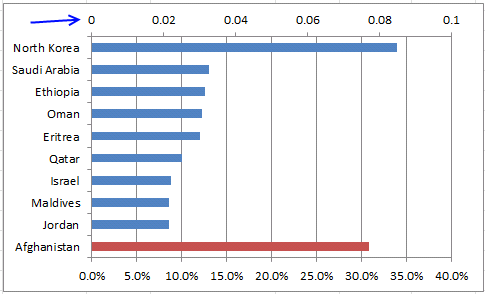2. Press Delete button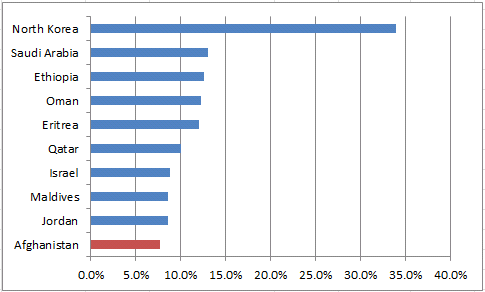#### Change bar color

1. Select a bar on the secondary axis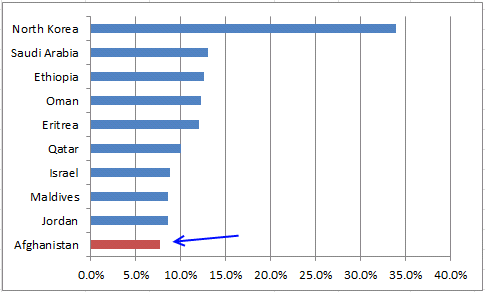2. Right click on bar
3. Click "Format Data Series..."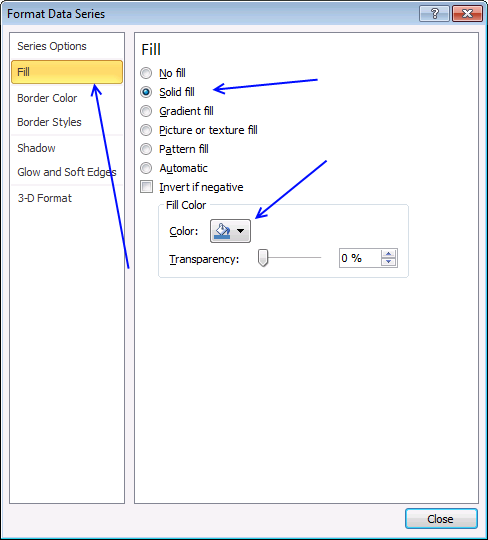4. Click "Fill"
5. Select "Solid Fill"
6. Select a color
7. Click Close

Repeat above steps with the second chartHighlight-a-bar-in-a-chart1.xlsm

### Highlight a bar in a chart (VBA)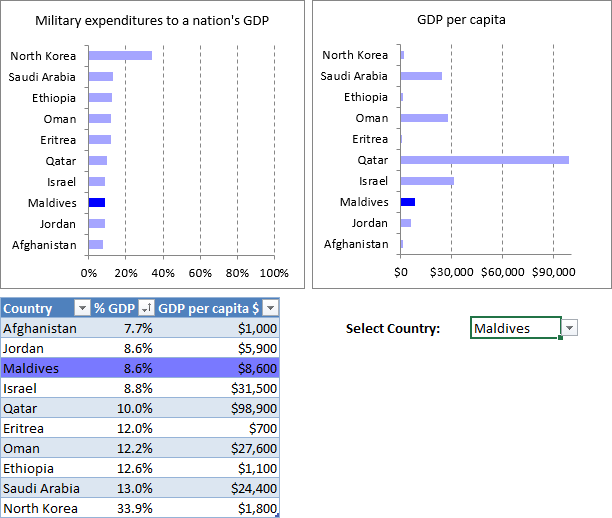I created two bar charts based on the data in Excel Table Table1, this VBA example has only one Excel Table. VBA code changes chart series color based on the selected value in cell H18.

#### Drop down list

1. Select cell H18
2. Go to tab "Data"
3. Click "Data Validation" button
4. Select List
5. Select table column Country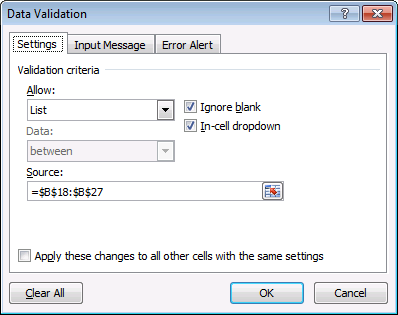1. Click OK

#### VBA Code

The following VBA code is event code, it is triggered when a cell value in a worksheet is changed. This code won't work if you have more than two charts inserted to the worksheet.

```'Event code
Private Sub Worksheet_Change(ByVal Target As Range)

'Dimension variables and declare data types
Dim r As Integer, chrtobj As Integer

'Check if cell is H18

'Iterate through 1 to 2
For chrtobj = 1 To 2

'Iterate through 1 to the number of countries in Table column Country
For r = 1 To Range("Table1[Country]").Rows.Count

'The With ... End With statement allows you to write shorter code by referring to an object only once instead of using it with each property.
With ActiveSheet.ChartObjects(chrtobj).Chart.SeriesCollection(1).Points(r).Interior

'Change chart series color to RGB(0, 0, 255) if row is equal to selected country
If Application.WorksheetFunction.Match(Range("H18"), Range("Table1[Country]"), 0) = r Then
.Color = RGB(0, 0, 255)
Else
.Color = RGB(165, 165, 255)
End If
End With
Next r
Next chrtobj
End If
End Sub
```

#### Where to put the code?

1. Right click on the sheet name and a context menu appears.
2. Click "View Code", see image below.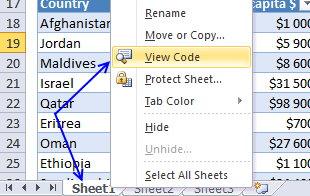3. Paste above VBA code to the sheet module. The image below shows that sheet1 is selected in the Project Explorer and the code window contains the VBA code.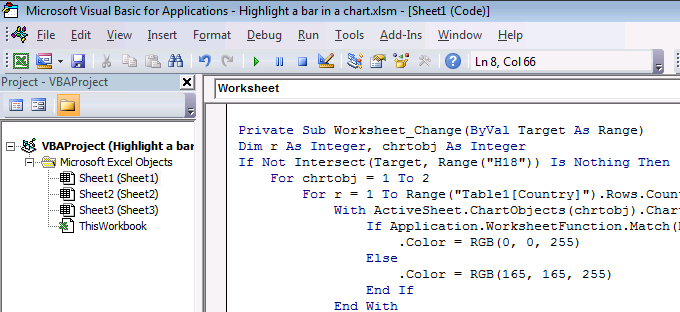#### Apply Conditional Formatting to Excel Table rows

1. Select table1.
2. Go to tab "Home" on the ribbon.
3. Click "Conditional formatting" button.
4. Click "New rule...".
5. Click "Use a formula to determine which cells to format".
6. Format values where this formula is true:
=INDIRECT("Table1[@Country]")=\$H\$18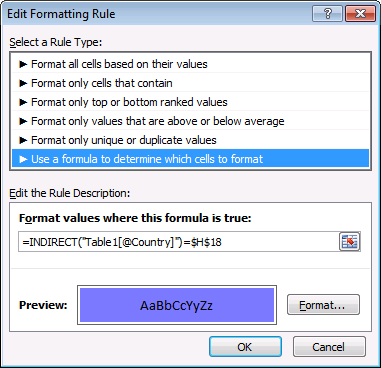7. Click "Format..." button
8. Go to tab "Fill"
9. Pick a color
10. Click OK
11. Click Ok